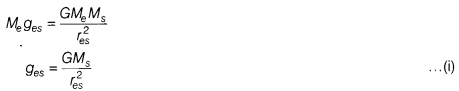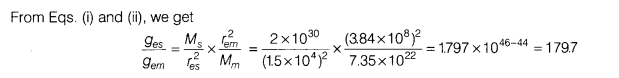# Calculate the ratio of the gravitational acceleration of the Earth due to the Sum with that of the Earth due to the Moon

Calculate the ratio of the gravitational acceleration of the Earth due to the Sum with that of the Earth due to the Moon. (Given, Me = 6 x 1024 kg, Ms = 2 x 1030 kg, Mm = 7.35 x 1022 kg, res = 1.5 x 1011 m and rem = 3.84 x 108 m)

Let the gravitational acceleration of the Earth due to the Sun is ges, we haveLet the gravitational acceleration of the Earth due to Moon is g, we have